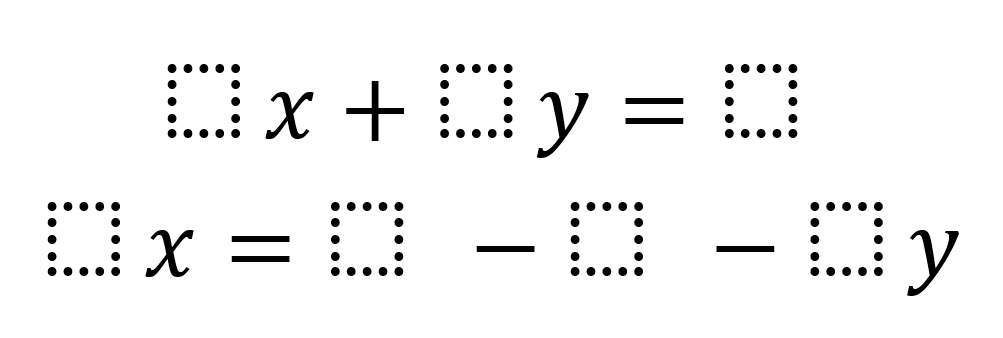# Infinitely Many Solutions System of Equations

Directions: Using the digits 1 to 9 at most one time each, place a digit in each box to create a a system of equations with infinitely many solutions.### Hint

What does it mean for a system of equations to have infinitely many solutions? How are the coefficients related in systems with infinitely many solutions?